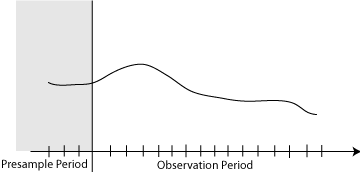Documentation

### This is machine translation

Mouseover text to see original. Click the button below to return to the English version of the page.

Note: This page has been translated by MathWorks. Click here to see
To view all translated materials including this page, select Country from the country navigator on the bottom of this page.

## Presample Data for Conditional Mean Model Estimation

Presample data comes from time points before the beginning of the observation period. In Econometrics Toolbox™, you can specify your own presample data or use generated presample data.In a conditional mean model, the distribution of εt is conditional on historical information. Historical information includes past responses, ${y}_{1},{y}_{2},\dots ,{y}_{t-1}$, past innovations, ${\epsilon }_{1},{\epsilon }_{2},\dots ,{\epsilon }_{t-1}$, and, if you include them in the model, past and present exogenous covariates, ${x}_{1},{x}_{2},\dots ,{x}_{t-1},{x}_{t}$.

The number of past responses and innovations that a current innovation depends on is determined by the degree of the AR or MA operators, and any differencing. For example, in an AR(2) model, each innovation depends on the two previous responses,

`${\epsilon }_{t}={y}_{t}-c-{\varphi }_{1}{y}_{t-1}-{\varphi }_{2}{y}_{t-2}.$`

In ARIMAX models, the current innovation also depends on the current value of the exogenous covariate (unlike distributed lag models). For example, in an ARX(2) model with one exogenous covariate, each innovation depends on the previous two responses and the current value of the covariate,

`${\epsilon }_{t}={y}_{t}-c-{\varphi }_{1}{y}_{t-1}-{\varphi }_{2}{y}_{t-2}+{x}_{t}.$`

In general, the likelihood contribution of the first few innovations is conditional on historical information that might not be observable. How do you estimate the parameters without all the data? In the ARX(2) example, ${\epsilon }_{2}$ explicitly depends on ${y}_{1},$ ${y}_{0},$ and ${x}_{2},$ and ${\epsilon }_{1}$ explicitly depends on ${y}_{0},$ ${y}_{-1},$ and ${x}_{1}$. Implicitly, ${\epsilon }_{2}$ depends on ${x}_{1}$ and ${x}_{0},$ and ${\epsilon }_{1}$ depends on ${x}_{0}$ and ${x}_{-1}.$ However, you cannot observe ${y}_{0},$ ${y}_{-1},$ ${x}_{0},$ and ${x}_{-1}.$

The amount of presample data that you need to initialize a model depends on the degree of the model. The property `P` of an `arima` model specifies the number of presample responses and exogenous data that you need to initialize the AR portion of a conditional mean model. For example, `P = 2` in an ARX(2) model. Therefore, you need two responses and two data points from each exogenous covariate series to initialize the model.

One option is to use the first `P` data from the response and exogenous covariate series as your presample, and then fit your model to the remaining data. This results in some loss of sample size. If you plan to compare multiple potential models, be aware that you can only use likelihood-based measures of fit (including the likelihood ratio test and information criteria) to compare models fit to the same data (of the same sample size). If you specify your own presample data, then you must use the largest required number of presample responses across all models that you want to compare.

The property `Q` of an `arima` model specifies the number of presample innovations needed to initialize the MA portion of a conditional mean model. You can get presample innovations by dividing your data into two parts. Fit a model to the first part, and infer the innovations. Then, use the inferred innovations as presample innovations for estimating the second part of the data.

For a model with both an autoregressive and moving average component, you can specify both presample responses and innovations, one or the other, or neither.

By default, `estimate` generates automatic presample response and innovation data. The software:

• Generates presample responses by backward forecasting.

• Sets presample innovations to zero.

• Does not generate presample exogenous data. One option is to backward forecast each exogenous series to generate a presample during data preprocessing.

Download ebook